# Kinetic Energy of Tube Flow

To get the kinetic energy of laminar flow in a tube, an average of the square of the velocity must be taken to account for the velocity profile.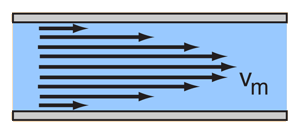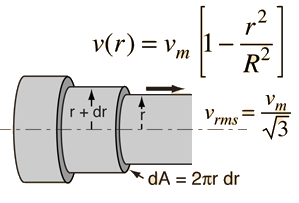The average of the square of the speed is given by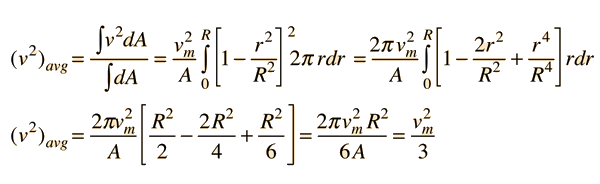The average kinetic energy per unit volume of the flowing fluid can be expressed in terms of the fluid density ρ and the maximum flow velocity vm.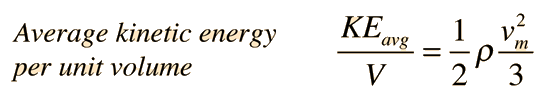Index

Poisuille's law concepts

 HyperPhysics***** Mechanics ***** Fluids R Nave
Go Back

# Velocity Relationship, Tube Flow

The nature of viscosity is such that successive lamina in the tube exert forces on each other according to the viscous force relationship: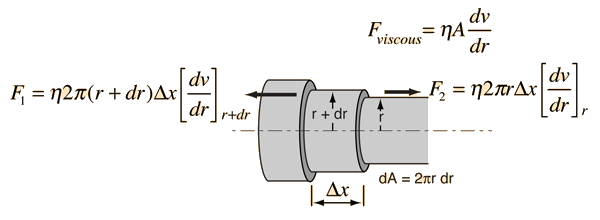When a pressure gradient dP/dx drives a section of lamina of length Δx at constant velocity, the force equation takes the form: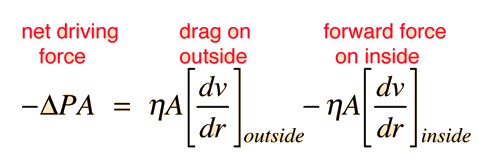For a short segment Δx of a given lamina, dA = 2πr dr and the forces take the form shown. This leads to an equation for the velocity in the tube.

 Development of velocity equation Equation solution Illustration of velocity profile.
Index

Poisuille's law concepts

 HyperPhysics***** Mechanics ***** Fluids R Nave
Go Back

# Velocity Equation, Tube Flow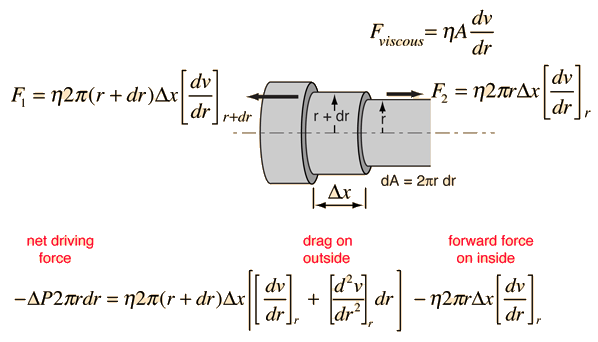Collecting terms gives the velocity equation in terms of radius r.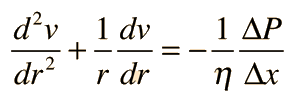Discussion of velocity equation Equation solution Illustration of velocity profile.
Index

Poisuille's law concepts

 HyperPhysics***** Mechanics ***** Fluids R Nave
Go Back

# Velocity Profile for Tube Flow

The relationship governing the velocity as a function of distance r from the center of a tube under conditions of laminar flow is:Show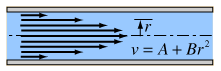Show details

The general form of the solution to this differential equation is v = A + Br2 where A and B are constants which must be fit to the boundary condition of the flow: v=0 at r=R. Substituting the general solution by taking the derivatives gives: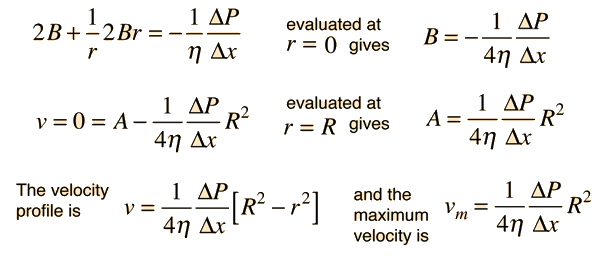Discussion of velocity equation Development of equation Illustration of velocity profile.
Index

Poisuille's law concepts

 HyperPhysics***** Mechanics ***** Fluids R Nave
Go Back

# Velocity Profile for Tube Flow

Under conditions of laminar flow, the nature of viscosity dictates a flow profile where the velocity increases toward the center of the tube as illustrated.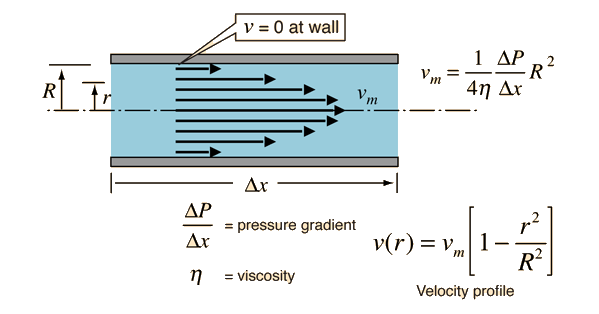Discussion of velocity equation Development of equation Equation solution
Index

Poisuille's law concepts

 HyperPhysics***** Mechanics ***** Fluids R Nave
Go Back